# T Distribution

• When do we use the T distribution

• Know the mean

• Do not know the population SD• Formula• Degrees of freedom (df)

• df = n-1

# Confidence Interval Example

The super Corporation manufactures a device they claim "may increase gas mileage by 23%"

Here are the percent changes in gas mileage for 17 identical vehicles, as presented in one of the company's advertisements

48.3 46.9 46.8 44.6 40.2 38.5
34.6 33.7 28.7 28.7 24.8 10.8
6.9 12.4 21.2 25.2

Construct and interpret a 90% confidence interval to estimate the mean fuel savings in the population of all such vehicles.

• Type in the data• 1-Var Stats for the sample• Looking for t• Calculate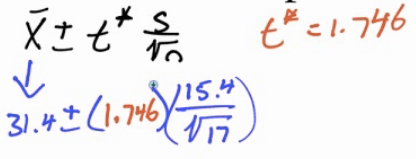• Calculate by calculator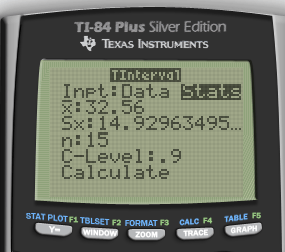• Interpret

• We are 90% confident that the mean percent change in gas mileage is between 24.68% and 38.16%

• Since 23% is in the interval, it appears that the machine does even better than 23% savings

# Hypothesis Test Example

When the manufacturing process is working properly, the batteries have lifetimes that follow a right-skewed distribution with µ = 10 hours. A quality control statistician selects a simple random sample of n = 20 batteries every hour and measures the lifetime of each.

If she is convinced that the mean lifetime of all batteries produced that hour is less than 10 hours at the 5% significance level, then all those batteries are discarded.

The current sample of 20 has a mean of 8.5 and a SD of 3, should this batch be discarded?

• Hypothesis

• H0: µ = 10

• H1: µ < 10

• Conditions

• Random: given

• Independent: N > 10n = 200

• Normal: not larger than 30, but still ok since we are using a T distribution

• Calculate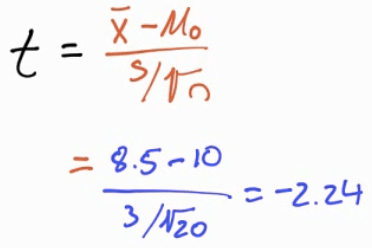• Calculate by calculator![Т1-И Pius Stver Editj(T ф TEns [NSTRUMENTS гоямдта сасса ](./media/image244.png)

• P-value• df = n - 1 = 19

• Interpret

• Because p < α, we reject the null hypothesis. Thus, we do have evidence to support the claim that the mean battery life in this whole batch is less than 10 hours and so should be discarded

# Matched Pairs T-Test

The average weekly loss of study hours due to consuming too much alcohol on the weekend is studied on 10 students before and after a certain alcohol aware ness program is put into operation. Do the data provide evidence that the program was effective?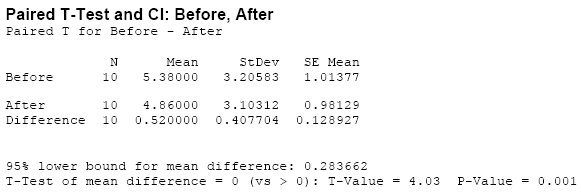• Formula• Data

• N=10

• Difference of mean = 0.52

• Difference of SD = 0.407

• Hypothesis (d = before - after)

• H0: µd = 0

• H1: µd > 0

• Conditions

• Random: assume random selection

• Independence: N > 10n = 100

• Normal: t-distribution

• Calculate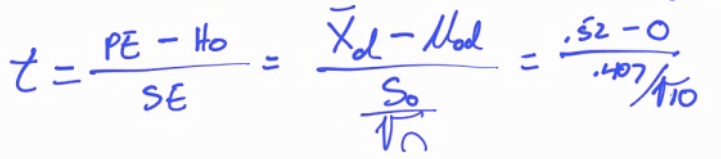• Calculate by calculator![ТИ-84 PIus Stver Editj(n ф TEns [NSTRUMENTS гоямдта сац:а ](./media/image250.png)

• P-value

• df = 10-1 = 9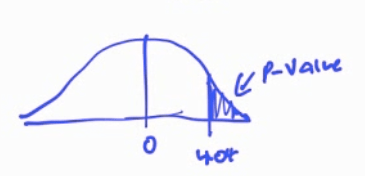• Interpret

• P < α, Reject, do have evidence